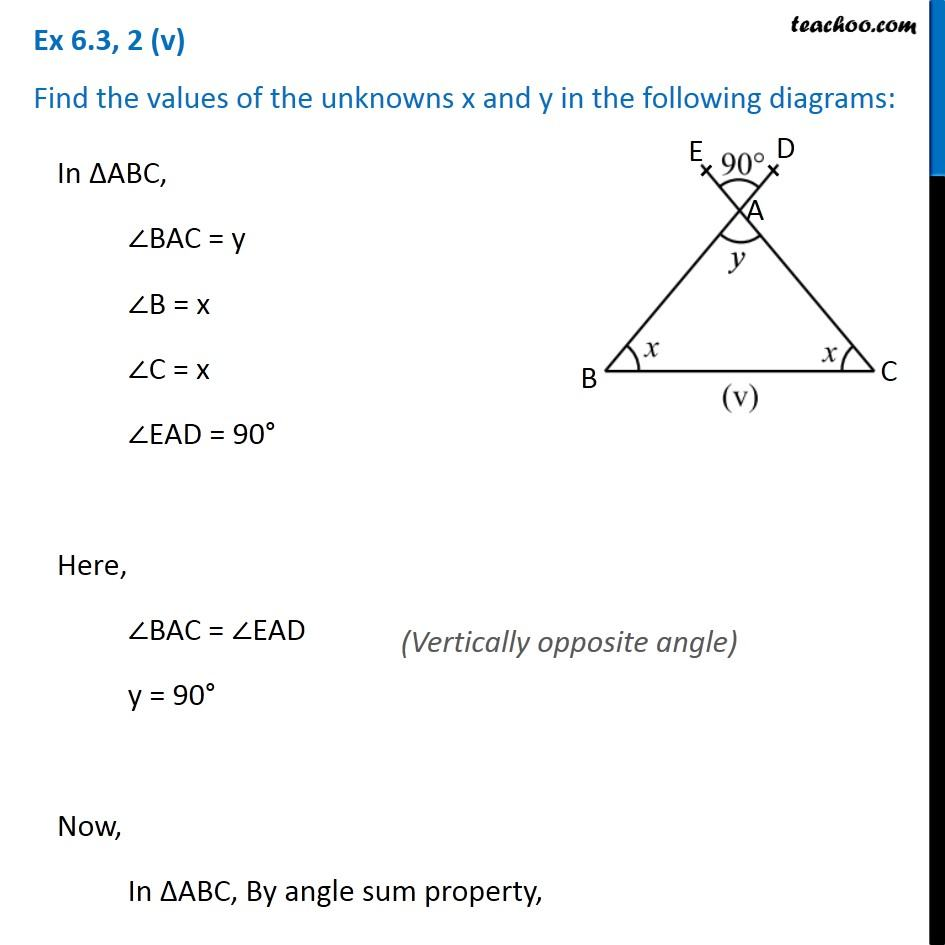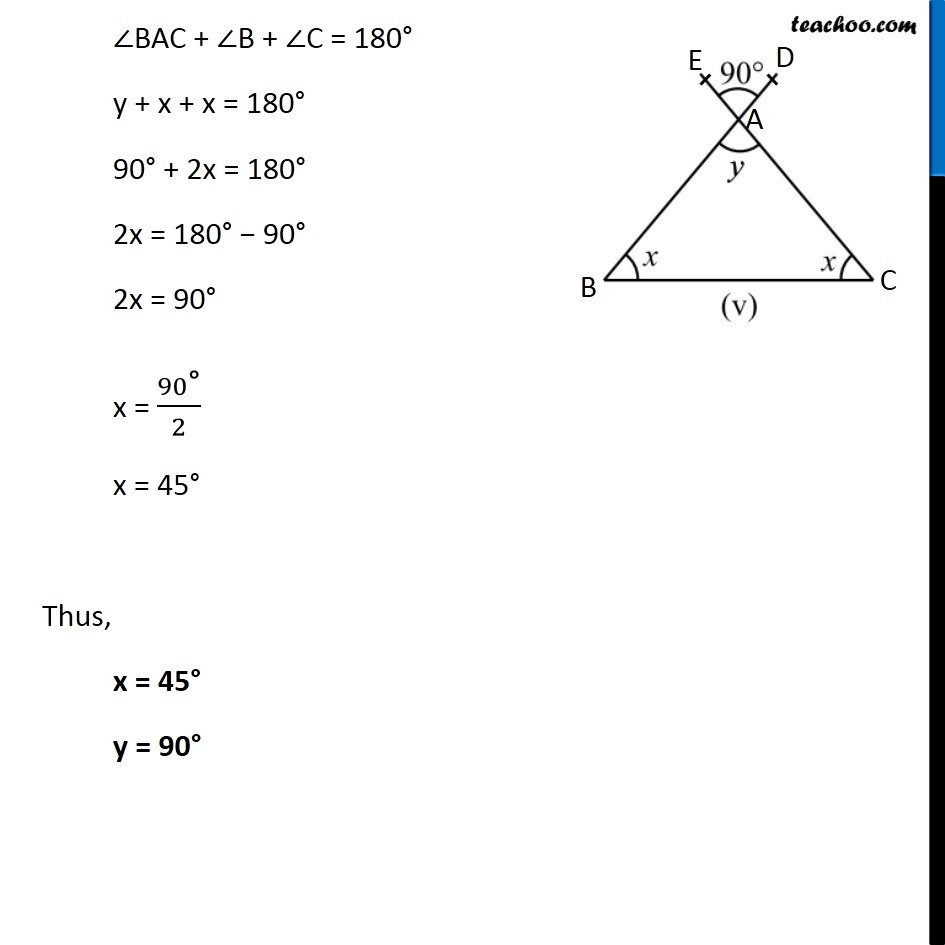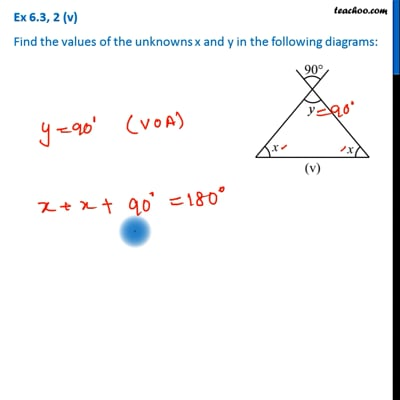Ex 6.3

Chapter 6 Class 7 Triangle and its Properties
Serial order wiseThis video is only available for Teachoo black users

Learn in your speed, with individual attention - Teachoo Maths 1-on-1 Class

### Transcript

Ex 6.3, 2 (v) Find the values of the unknowns x and y in the following diagrams: In ∆ABC, ∠BAC = y ∠B = x ∠C = x ∠EAD = 90° Here, ∠BAC = ∠EAD y = 90° Now, In ∆ABC, By angle sum property, ∠BAC + ∠B + ∠C = 180° y + x + x = 180° 90° + 2x = 180° 2x = 180° − 90° 2x = 90° x = 90"°" /2 x = 45° Thus, x = 45° y = 90°